# Geometry Practice Test 2

##### 1. Given the triangle shown in the figure, what is the length of the side A?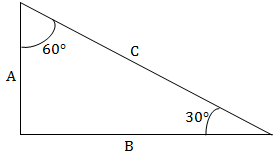1. C/2
2. A/2
3. (A+C)/2
4. 2A
5. 2C
##### 2. A circle is inscribed within a square, as shown. What is the difference between the area of the square and that of the circle, where r is the radius of the circle?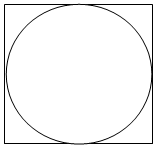1. 2πr2
2. 4/3 πr3
3. r2 (4-π)
4. r
5. 2r2
1. 90 degrees
2. 80 degrees
3. 75 degrees.
4. 125 degrees
5. 95 degrees
1. 20
2. 30
3. 40
4. 50
5. 60
##### 5. The diagram shows the outline of a racetrack for skaters, which consists of two long straight sections and two semi-circular turns. Given the dimensions shown, which of the following most closely measures the perimeter of the entire track?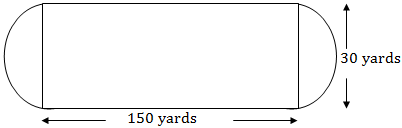1. 180 yards
2. 360 yards
3. 395 yards
4. 425 yards
##### 6. A tire on a car rotates at 500 RPM (revolutions per minute) when the car is traveling at 50 km/hr (kilometers per hour). What is the circumference of the tire, in meters?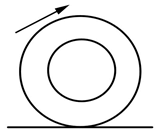1. 50,000/2π
2. 50,000/(60*2π)
3. 50,000/(500*2π)
4. 50,000/60
5. 10/6
1. πr2
2. πr2/2π
3. 2πr/r2
4. 2πr1/2
5. r/2
##### 8. Lines AC and BD intersect at point E. Angle BEC is 450. What is the measure of angle AEB?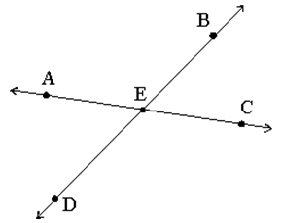1. Angle AEB is 900
2. Angle AEB is 1150
3. Angle AEB is 1350
4. Angle AEB is 1800
5. Angle AEB is 3600
1. 710 and 190
2. 180 and 180
3. 900 and 900
4. 900 and 450
5. 150 and 300
##### 10. Which of the following letters has a vertical line of symmetry?
1. A
2. B
3. C
4. D
5. E

###### 1. A

Since the two angles shown add up to 90 degrees, and the remaining angle must therefore be 90 degrees, this is a right triangle. For a right triangle, the length of a side is related to the hypotenuse by the sine of the opposite angle. Thus, A=Csin(300) and since the sine of a 30-degree angle is 0.5 or 1/2, A=C/2 .

###### 2. C

The side of the square is equal to the diameter of the circle, or twice the radius: 2r. The area of the square is this quantity 2r squared, or 4r2. The area of the circle is πr2. Subtracting gives the difference between the two areas: 4r2-πr2=r2 (4-π)

###### 3. E

The sum of angles in a triangle equals 180 degrees. Therefore, solve for the remaining angle by subtracting the sum of the two given angles from 180 degrees: 180 – (15 + 70) = 95 degrees.

4. B

The perimeter of a square is four times the length of any one of its sides. If a square’s perimeter is 20, the length of any side is 5. The perimeter of the rectangle described in this problem is six times the length of a side of the square, which is 6*5=30.

###### 5. C

First, add the two straight 150 yard portions. Also, note that the distance around the two semi-circle turns combine to form the circumference of a circle. The radius (r) of that circle is half of the dimension shown as the width of the track, or 15 yards. Now, taking the formula for the circumference of a circle, 2πr (with r = 15), and adding it to the length of the two straight portions of the track, we have:Length=(2π*15)+(2*150)=394.25.

###### 6. E

It is not necessary to use the circle circumference formula to solve the problem. Rather, note that 50 km/hr corresponds to 50,000 meters per hour. We are given the car tire’s revolutions per minute and the answer must be represented as meters; therefore, the speed must be converted to meters per minute. This corresponds to a speed of meters 50,000/60 per minute, as there are 60 minutes in an hour. In any given minute, the car travels 50,000/60 meters/min, and each tire rotates 500 times around, or 500 times its circumference. This corresponds to 50,000/(60*500)=10/6 meters per revolution, which is the circumference of the tire.

###### 7. E

The area of the circle is πr2 while the circumference is 2πr. Taking the ratio of these two expressions and reducing gives: io=(πr2)/2πr=r/2.

###### 8. C

Note that angles AEB and BEC are supplementary and so their sum is 1800. Subtract to solve: 1800 – 450 = 1350.

###### 9. A

Complementary angles are two angles that equal 900 when added together.

###### 10. A

If you draw a vertical line down the center of the letter A, the two sides will be symmetrical.